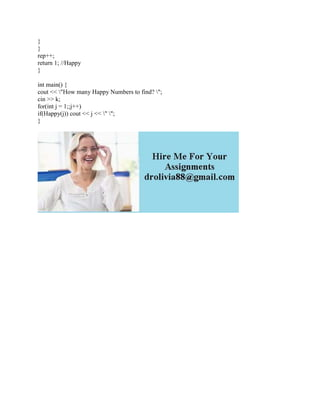Ce diaporama a bien été signalé.
Le téléchargement de votre SlideShare est en cours. ×

Publicité
Publicité
Publicité
Publicité
Publicité
Publicité
Publicité
Publicité
Publicité
Publicité
PublicitéChargement dans…3
×

## Consultez-les par la suite

1 sur 2 Publicité

Part 2: happy.cpp Write a C++ program happy.cpp which prints the first 88,888 happy numbers The numbers should be printed 10 per line, with a single space between the values. The last line should only have 8 numbers on it. The first line of the output should be 1 7 10 13 19 23 28 31 32 44 See wikipedia or wolfram for the definition of a happy number.
Solution
//infinite happy number\'s
#include <iostream>
#include <cmath>
#include <cstdlib>
using namespace std;
int k, rep = 0;
int SumOfSq(int num) {
int total = 0;
while(num) {
int digit = num % 10;
num /= 10;
total += pow(digit,2);
}
}
bool Happy(int num) {
int temp = 0;
while(num != 1) {
if(k == rep) exit(1);
num = SumOfSq(num);
if(temp++ > 10) {
return 0; //Not happy
}
}
rep++;
return 1; //Happy
}
int main() {
cout << \"How many Happy Numbers to find? \";
cin >> k;
for(int j = 1;;j++)
if(Happy(j)) cout << j << \" \";
}
.

Part 2: happy.cpp Write a C++ program happy.cpp which prints the first 88,888 happy numbers The numbers should be printed 10 per line, with a single space between the values. The last line should only have 8 numbers on it. The first line of the output should be 1 7 10 13 19 23 28 31 32 44 See wikipedia or wolfram for the definition of a happy number.
Solution
//infinite happy number\'s
#include <iostream>
#include <cmath>
#include <cstdlib>
using namespace std;
int k, rep = 0;
int SumOfSq(int num) {
int total = 0;
while(num) {
int digit = num % 10;
num /= 10;
total += pow(digit,2);
}
}
bool Happy(int num) {
int temp = 0;
while(num != 1) {
if(k == rep) exit(1);
num = SumOfSq(num);
if(temp++ > 10) {
return 0; //Not happy
}
}
rep++;
return 1; //Happy
}
int main() {
cout << \"How many Happy Numbers to find? \";
cin >> k;
for(int j = 1;;j++)
if(Happy(j)) cout << j << \" \";
}
.

Publicité
Publicité